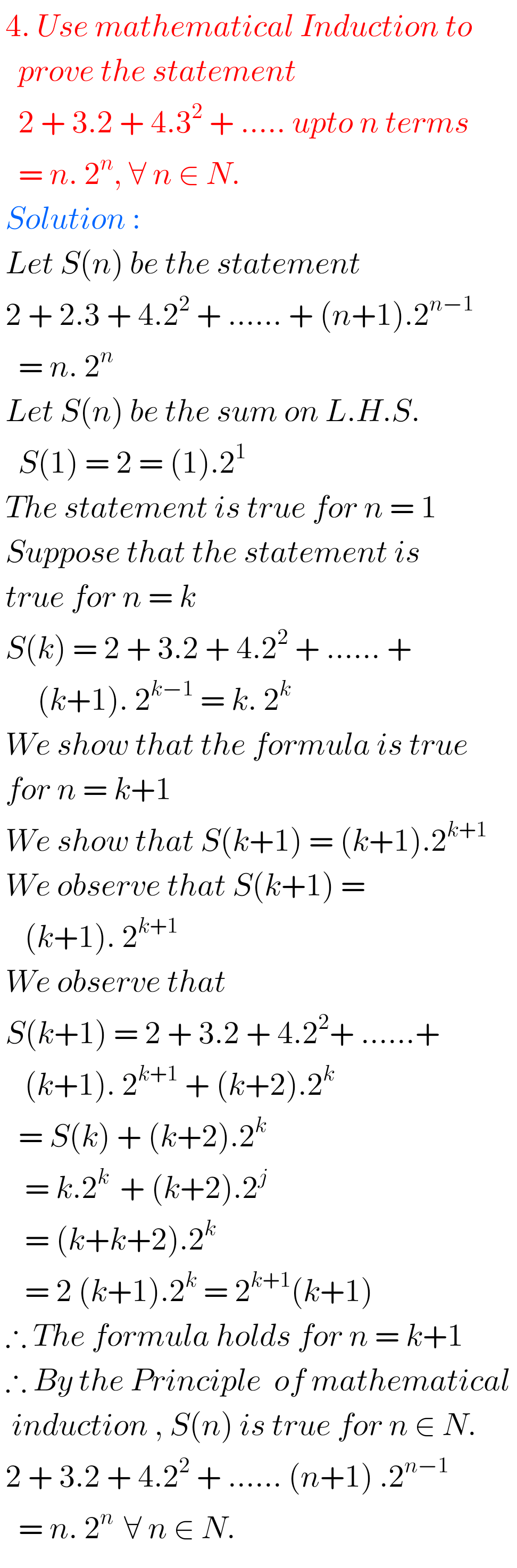# worked examples mathematical induction## MATHEMATICAL INDUCTION, Intermediate 1st year problems with solutions,solutions for Mathematical Induction Inter first year 1A

MATHEMATICAL INDUCTION, INTERMEDIATE FIRST YEAR PROBLEMS WITH SOLUTIONS Mathematics Intermediate first year 1A Mathematical Induction solutions for some problems.  These solutions are very simple to understand.  Study the textbook lesson Mathematical Induction very well  Observe the example problems and solutions given in the textbook  You can also see Inter Maths 1A textbook solutions Inter Maths …

## NCERT solutions for class 11th maths chapter 4,Principle of Mathematical Induction

Principle of Mathematical Induction solutions class 11 Ncert CBSE solutions for class 11 Pribciple of Mathematical Induction solutions are given in pdf Principle of Mathematical induction solutions Ncert Mathematical induction solutions for inter Functions solutions for intermediate Properties of triangles solutions for intermediate Trigonometric ratios upto transformations solutions intermediate.  Trigonometric equations solutions intermediate Product of …